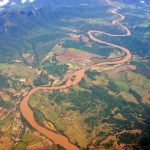# R FOR HYDROLOGISTS: Correlation and Information Theory Measurements – Part 2: Exercises

March 27, 2018
By

Want to share your content on R-bloggers? click here if you have a blog, or here if you don't.R FOR HYDROLOGISTS

CORRELATION AND INFORMATION THEORY MEASUREMENTS (Part 2)

Proposed back in the 40’s by Shannon Information theory provide a framework for the analysis of randomness in time-series, and information gain when comparing statistical models of inference. Information theory is based on probability theory and statistics. It often concerns itself with measures of information of the distributions associated with random variables. Important quantities of information are Entropy, a measure of information in a single random variable, Mutual information, a measure of information in common between two random variables, and Relative entropy that measure how one probability distribution diverges from a second expected probability distribution.

In this tutorial we will estimate these measurements in order to characterize the river dynamic. If you don’t have the data please first see the first part of the tutorial here and Install and load `ggplot2` and `reshape2` packages
``` if(!require(ggplot2)){install.packages(ggplot2, dep=T)} if(!require(reshape2)){install.packages(reshape2, dep=T)} ```
Answers to the exercises are available here.

All information measurements are derivate from the join and marginal distributions of two variables. To estimate this empiric distribution we will use histograms; in this opportunity we will `geom_bin2d`. Let’s do it step by step.

Exercise 1
First please create a `geom_point` plot of the `LEVEL` against the ` RAIN`

Exercise 2
Now please overlap a 2D histogram with the function `geom_bin2d()`

Exercise 3
We have to get the joint probability matrix. So please set the number of `bins =10` and plot the joint probability distribution of the `LEVEL` and the ` RAIN ` then assign it to an object `p`.

Exercise 4
Extract from the object p the data of the first layer with the function `layer_data` and assign it to `pxy_m`.

Exercise 5
As you can see `ggplot` return a column based data frame with the `x`, `y` and the value of the density index as columns. Please convert it to a rectangular matrix with the function `acast` and sign it to `pxy`

Exercise 6
Please guarantee the natural restriction the probability distribution `sum(pxy)==1`

Learn more about Model Evaluation in the online course Regression Machine Learning with R. In this course you will learn how to:

• Avoid model over-fitting using cross-validation for optimal parameter selection
• use correlation to avoid multi-collinearity problems in your model.
• And much more

Exercise 7
Estimate the marginal probabilities `px` and `py`.

Exercise 8
Great now we have everything we need. Please estimate the entropy in bits (log2) for each variable `Hx` and `Hy`.

Exercise 9

Estimate the Joint entropy in bits (log2) with the formula: `Hxy=-sum(pxy*log2(pxy))`. Remember that in order to avoid numerical error you have to use just positives probabilities pxy>0 before applying the formula

Exercise 10
Last step, please calculate the mutual information Hint: `MI=Hx+Hy-Hxy`

R-bloggers.com offers daily e-mail updates about R news and tutorials about learning R and many other topics. Click here if you're looking to post or find an R/data-science job.
Want to share your content on R-bloggers? click here if you have a blog, or here if you don't.